Скачать презентацию APPENDIX Present Value and Discounting 17 After

3599a05f952ef387e4ac0e6b37e4d013.ppt

• Количество слайдов: 16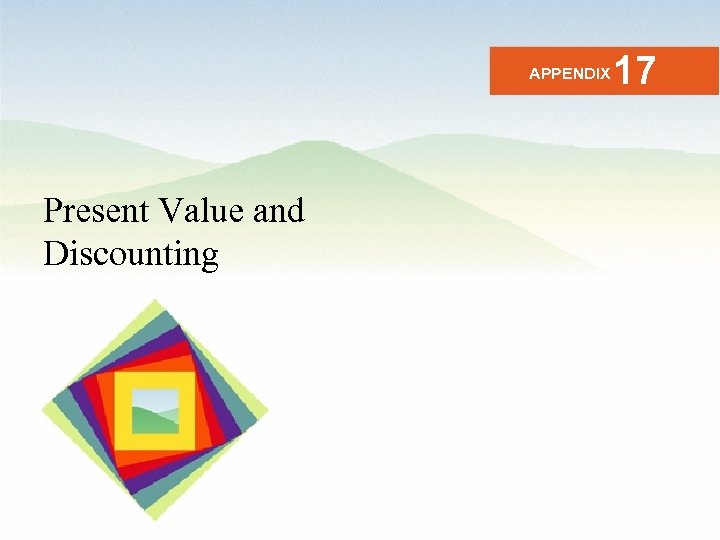APPENDIX Present Value and Discounting 17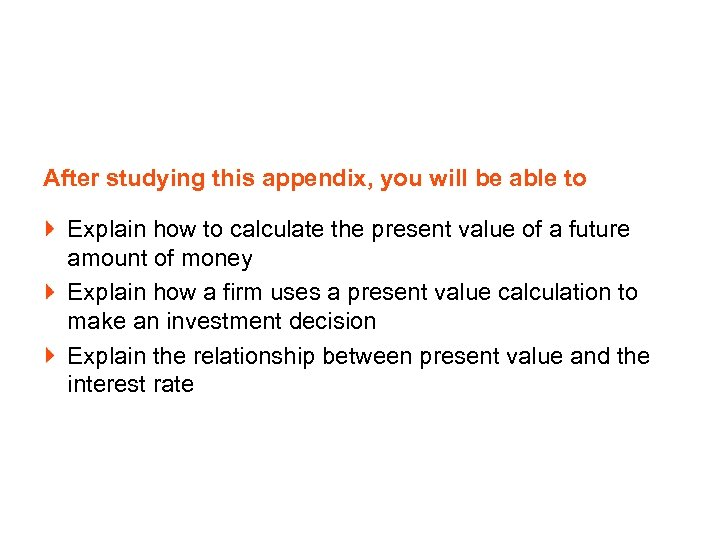After studying this appendix, you will be able to Explain how to calculate the present value of a future amount of money Explain how a firm uses a present value calculation to make an investment decision Explain the relationship between present value and the interest rate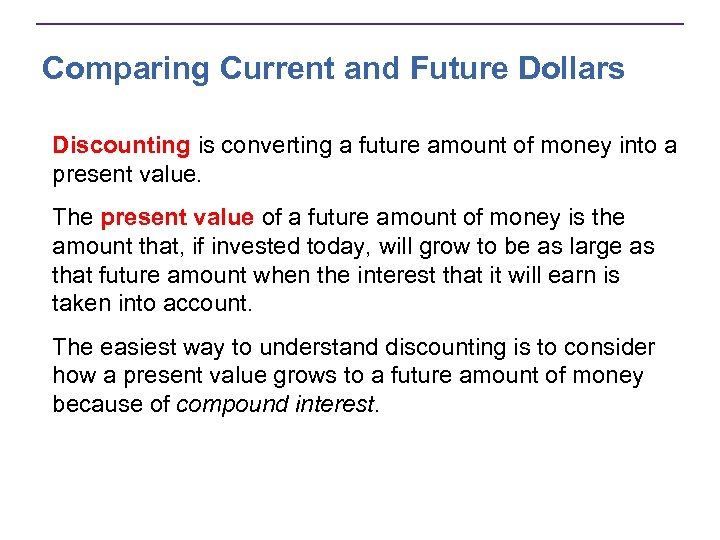Comparing Current and Future Dollars Discounting is converting a future amount of money into a present value. The present value of a future amount of money is the amount that, if invested today, will grow to be as large as that future amount when the interest that it will earn is taken into account. The easiest way to understand discounting is to consider how a present value grows to a future amount of money because of compound interest.Comparing Current and Future Dollars Compound Interest Compound interest is the interest on an initial investment plus the interest on the interest that the investment has previously earned. Because of compound interest, a present amount of money (a present value) grows into a larger future amount. Future amount = Present value + Interest income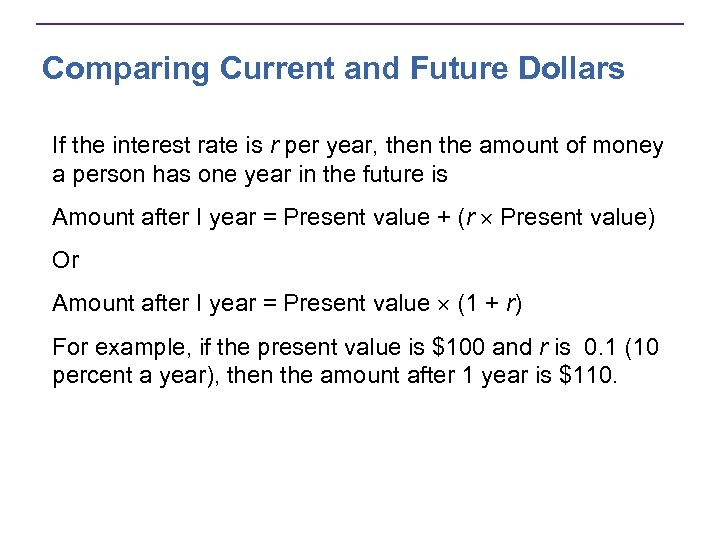Comparing Current and Future Dollars If the interest rate is r per year, then the amount of money a person has one year in the future is Amount after I year = Present value + (r Present value) Or Amount after I year = Present value (1 + r) For example, if the present value is \$100 and r is 0. 1 (10 percent a year), then the amount after 1 year is \$110.Comparing Current and Future Dollars After 2 years: Amount after 2 years = Present value (1 + r)2 For example, if the present value is \$100 and r is 0. 1 (10 percent a year), then Amount after 2 years = \$100 (1 + 0. 1)2 Amount after 2 years = \$100 (1. 1)2 Amount after 2 years = \$121 Similarly, the amount of money after n years is Amount after n years = Present value (1 + r)n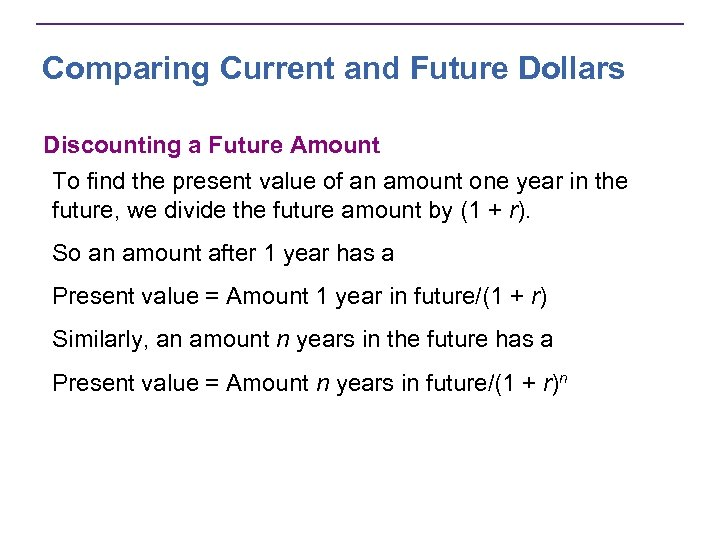Comparing Current and Future Dollars Discounting a Future Amount To find the present value of an amount one year in the future, we divide the future amount by (1 + r). So an amount after 1 year has a Present value = Amount 1 year in future/(1 + r) Similarly, an amount n years in the future has a Present value = Amount n years in future/(1 + r)n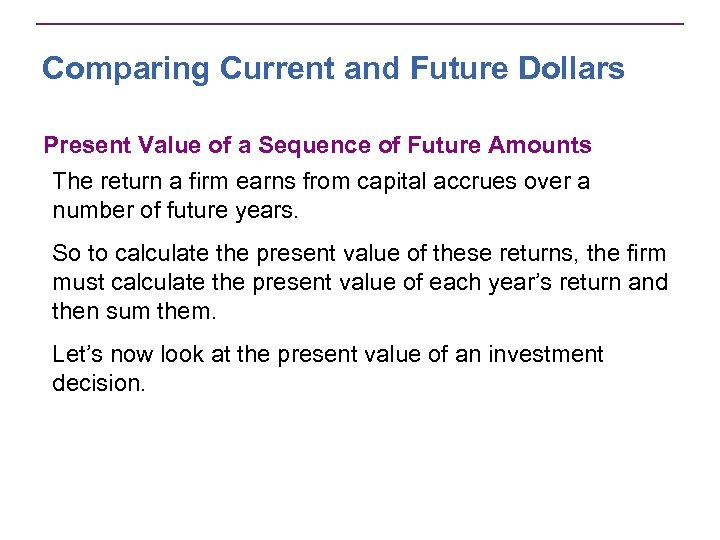Comparing Current and Future Dollars Present Value of a Sequence of Future Amounts The return a firm earns from capital accrues over a number of future years. So to calculate the present value of these returns, the firm must calculate the present value of each year’s return and then sum them. Let’s now look at the present value of an investment decision.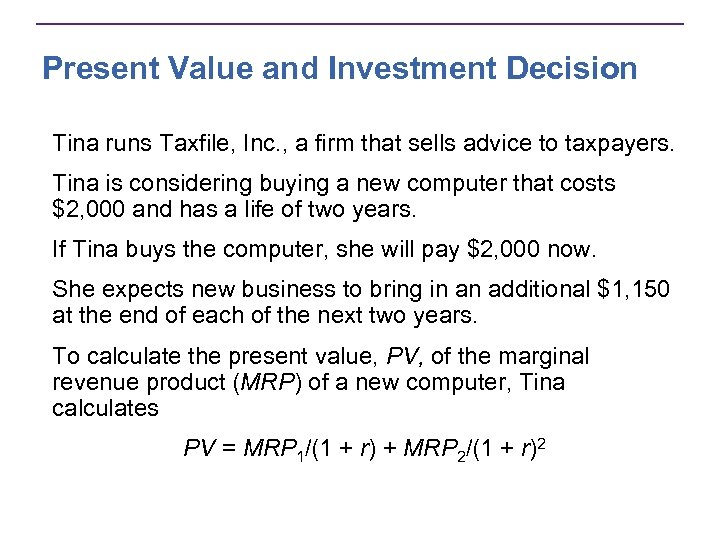Present Value and Investment Decision Tina runs Taxfile, Inc. , a firm that sells advice to taxpayers. Tina is considering buying a new computer that costs \$2, 000 and has a life of two years. If Tina buys the computer, she will pay \$2, 000 now. She expects new business to bring in an additional \$1, 150 at the end of each of the next two years. To calculate the present value, PV, of the marginal revenue product (MRP) of a new computer, Tina calculates PV = MRP 1/(1 + r) + MRP 2/(1 + r)2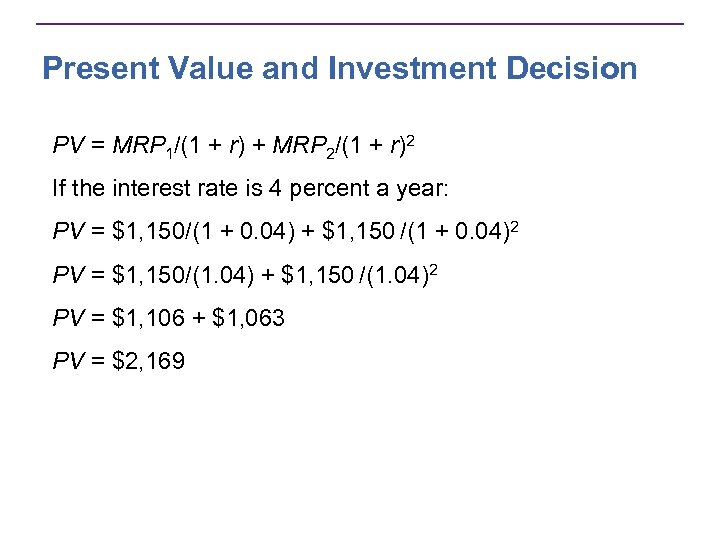Present Value and Investment Decision PV = MRP 1/(1 + r) + MRP 2/(1 + r)2 If the interest rate is 4 percent a year: PV = \$1, 150/(1 + 0. 04) + \$1, 150 /(1 + 0. 04)2 PV = \$1, 150/(1. 04) + \$1, 150 /(1. 04)2 PV = \$1, 106 + \$1, 063 PV = \$2, 169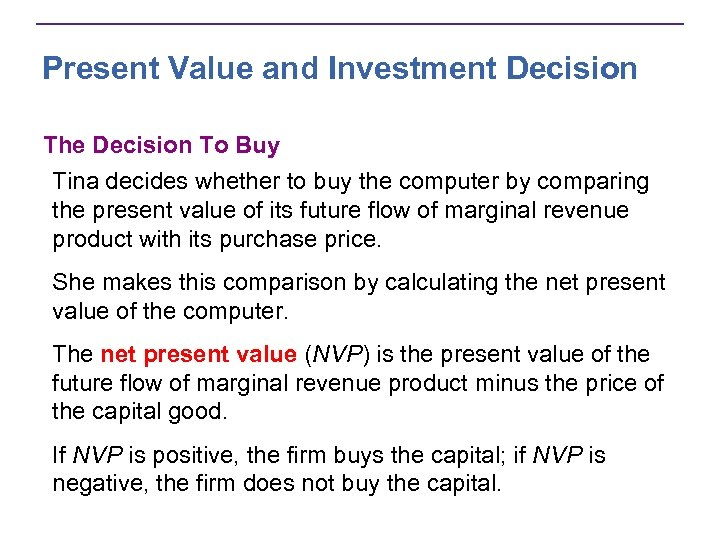Present Value and Investment Decision The Decision To Buy Tina decides whether to buy the computer by comparing the present value of its future flow of marginal revenue product with its purchase price. She makes this comparison by calculating the net present value of the computer. The net present value (NVP) is the present value of the future flow of marginal revenue product minus the price of the capital good. If NVP is positive, the firm buys the capital; if NVP is negative, the firm does not buy the capital.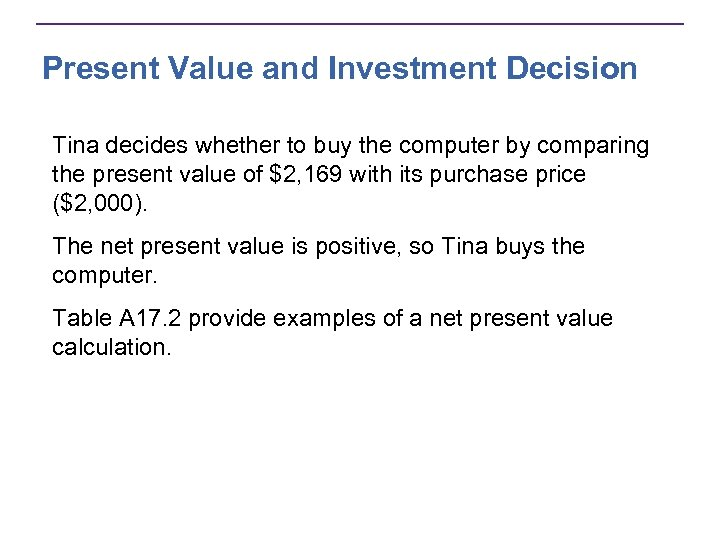Present Value and Investment Decision Tina decides whether to buy the computer by comparing the present value of \$2, 169 with its purchase price (\$2, 000). The net present value is positive, so Tina buys the computer. Table A 17. 2 provide examples of a net present value calculation.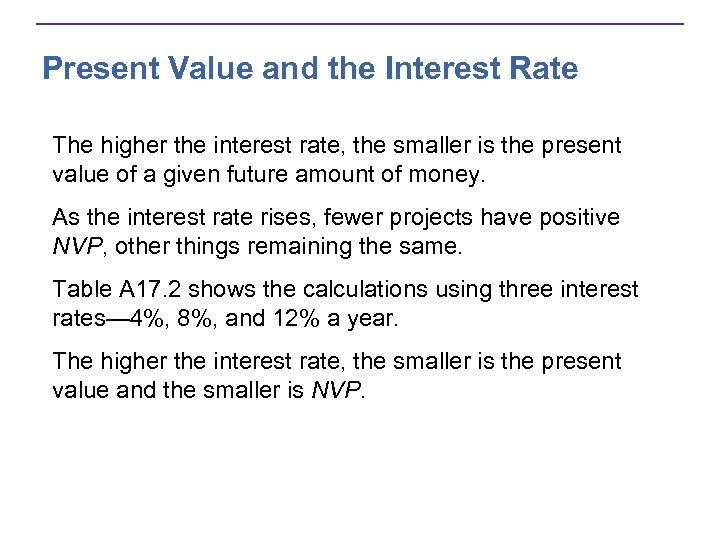Present Value and the Interest Rate The higher the interest rate, the smaller is the present value of a given future amount of money. As the interest rate rises, fewer projects have positive NVP, other things remaining the same. Table A 17. 2 shows the calculations using three interest rates— 4%, 8%, and 12% a year. The higher the interest rate, the smaller is the present value and the smaller is NVP.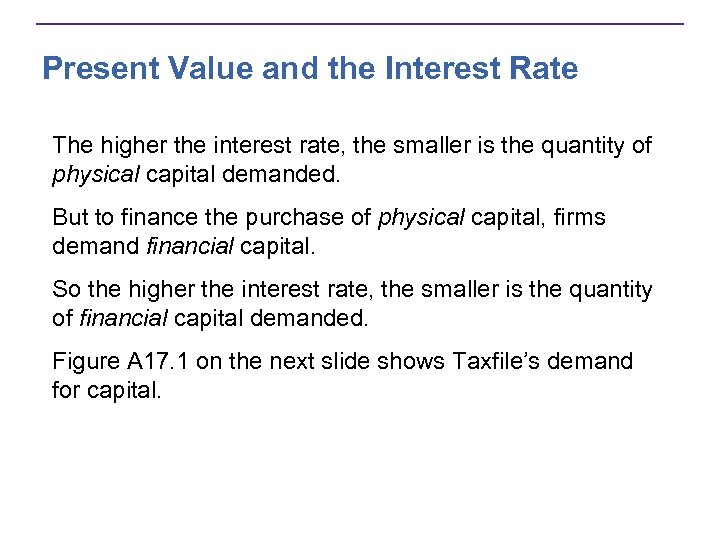Present Value and the Interest Rate The higher the interest rate, the smaller is the quantity of physical capital demanded. But to finance the purchase of physical capital, firms demand financial capital. So the higher the interest rate, the smaller is the quantity of financial capital demanded. Figure A 17. 1 on the next slide shows Taxfile’s demand for capital.Present Value and the Interest Rate Figure A 17. 1 shows a firm’s demand curve for capital. This demand curve is based on the calculations in Table A 17. 2.THE END